﻿ Graph Theoretical Models of Discrete Spaces with Locally Non-spherical TopologyPublications are Open
Access in this journal
Article Versions
Export Article
• Normal Style
• MLA Style
• APA Style
• Chicago Style
Research Article
Open Access Peer-reviewed

### Graph Theoretical Models of Discrete Spaces with Locally Non-spherical Topology

Alexander V. Evako
Applied Mathematics and Physics. 2017, 5(2), 47-52. DOI: 10.12691/amp-5-2-3
Published online: May 20, 2017

### Abstract

A graph theoretical model of a continuous space is a graph with the same topological structure as its continuous counterpart. A digital closed n-dimensional manifold with a locally spherical topology is a graph theoretic model for a continuous closed n-dimensional manifold. This paper defines and studies properties of a new class of digital n-dimensional spaces with a locally non-spherical topology. We prove that such spaces have the dimension n≥3. We define and investigate properties of digital 3- and 5-dimensional closed surfaces with a local toroidal and projective plane topology. These spaces have no direct continuous counterparts among n-dimensional manifolds in classical topology. These results arise questions like what physical, chemical or biological structures can be described by digital n-dimensional surfaces with a locally non-spherical topology.

### 1. Introduction

This paper studies the structure of graphs that are digital n-dimensional spaces with a locally spherical and locally non-spherical topology. Digital n-dimensional spaces with a locally spherical topology are graph theoretical models of continuous n-dimensional spaces as it is shown in 7, 11. We discuss the particular case when the graph is a digital n-dimensional surface with a locally non-spherical topology.

The key issue raised by this paper is what physical, chemical or biological structures are that can be represented by graphs that are digital n-dimensional manifolds with a locally non-spherical topology.

Constructing and analyzing n-dimensional discrete and digitized images of continuous objects is central to many applications: physics, geoscience, computer graphics, fluid dynamics and medicine 16 are obvious examples.

In physics, an abundant number of papers is devoted to the study of structures and processes involving graphs and non-orientable spaces.

A a lattice structure of a nucleus is studied in many theoretical models. A review of works devoted to the structures of nuclei as the result of octahedron shaped protons and neutrons can be found in 1.

Postulating that physical space is described by a 3-dimensional cubic lattice, the author introduces and investigates the elementary particle cube that is the graph with 27 adjacent points, and considers its points as pre-elementary particles (see 12). Each elementary particle is described by a unique subgraph of the graph.

In 15, numerical methods are used in the study of lattice models on non-orientable surfaces such as a Moebius strip and a Klein bottle. Graph theoretical and combinatorial models for continuous physical spaces were considered in 10.

Since analytic solutions of many problems arising in theories involving continuous objects in general and non-orientable surfaces in particular can be obtained only on simple geometric regions, it is crucial to replace continuous objects with mathematically correct discrete models that allows to apply computational or numerical methods.

There are a number of different frameworks to represent discrete models of continuous objects. Graph-theoretic approach equips a digital image with a graph structure based on the local adjacency relations of points (see e.g. 2, 3, 4). In paper 4, digital n-surfaces were defined as simple undirected graphs and basic properties of n-surfaces were studied. Properties of digital n-manifolds were investigated in 5, 6, 9, 10.

Paper 11 proves that the intersection graph of any LCL cover of a continuous closed n-dimensional manifold is necessarily a digital n-dimensional manifold preserving global and local topological features of its continuous counterpart.

The present paper is organized as follows. Section 2 describes properties of contractible graphs, homotopy equivalent graphs and contractible transformations.

Sections 3 and 4 summarize necessary results related to the structure of graphs that are digital n-dimensional surfaces, spheres and manifolds, and to the homeomorphism of graphs as discrete spaces..

In section 5, we define digital n-dimensional surfaces with a locally non-spherical topology, and consider difference between digital n-dimensional surfaces with a locally spherical and locally non-spherical topology. We study properties of digital n-dimensional surfaces with a locally non-spherical topology and show that the dimension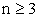. The examples are given of digital n-dimensional surfaces with a local toroidal topology and local projective plane topology.

Our results could easily be applied to analyze the topological structure of atoms, molecules and any objects having a discrete basis. These results arise questions like what physical, chemical or biological structure can be described by digital n-dimensional surfaces with a locally non-spherical topology.

### 2. Contractible Graphs, Homotopy Equivalent Graphs and Contractible Transformations

In order to make this paper self-contained we will summarize the necessary information from previous papers. Traditionally, a digital image has a graph structure (see 2, 3, 4). A digital space G is a simple undirected graph G=(V, W) where V=(v1, v2, ... vn, …) is a finite or countable set of points, and W = ((vрvq),....) is a set of edges. Topological properties of G as a digital space in terms of adjacency, connectedness and dimensionality are completely defined by set W. Let G and v be a graph and a point of G. In ( 13, 14), the subgraph O(v) containing all neighbors of v (without v) is called the rim of point v in G. The subgraph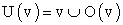containing O(v) as well as point v is called the ball of point v in G. Let (vu) be an edge of G. The subgraph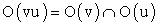is called the rim of (vu).

For two graphs G=(X, U) and H=(Y, W) with disjoint point sets X and Y, their join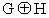is the graph that contains G, H and edges joining every point in G with every point in H.

Contractible graphs and contractible transformations are basic elements in this approach (see 13, 14).

Definition 1

• A one-point graph is contractible. If G is a contractible graph and H is a contractible subgraph of G then G can be converted into H by sequential deleting simple points.

• A point v in graph G is simple if the rim O(v) of v is a contractible graph.

• An edge (uv) of a graph G is called simple if the rimof (uv) is a contractible graph.

By construction, a contractible graph is connected. It follows from definition 1 that a contractible graph can be converted to a point by sequential deleting simple points. Figure 1 depicts contractible graphs with the number of points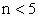.

Definition 2

• Deletions and attachments of simple points and edges are called contractible transformations.

• Graphs G and H are called homotopy equivalent if one of them can be converted to the other one by a sequence of contractible transformations.

Homotopy is an equivalence relation among graphs. Contractible transformations of graphs seem to play the same role in this approach as a homotopy in algebraic topology. In papers 13, 14, it was shown that contractible transformations retain the Euler characteristic and homology groups of a graph. All graphs depicted in Figure 1 are homotopy equivalent, i. e., any graph can be converted to a one-point graph G1 by contractible transformations.

### 3. Digital n-dimensional Surfaces and Homeomorphism

This section includes results obtained in 4, 5, 6, 10, 13, 14 and related to the structure of graphs that are digital n-dimensional spaces which were defined in 4.

Definition 3

The digital 0-dimensional surface S0(a, b) is a disconnected graph with just two points a and b. For n>0, a digital n-dimensional surface G is a nonempty connected graph such that for each point v of Gn, the rim O(v) is a finite digital (n-1)-dimensional surface.

S0(a, b) is called a digital 0-sphere (Figure 2). Digital 1-surfaces G1, G2 and G3 are shown in Figure 2. The following theorem provides a method of obtaining higher-dimensional surfaces from lower-dimensional ones. It was proven in 4. Here, we give a new proof of this theorem.

Theorem 1

Let G and H be digital n- and m-dimensional surfaces. Then the join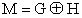is a digital (n+m+1)-dimensional surface.

Proof

The proof is by induction on m+n. For n+m=0,1, the theorem is plainly true (see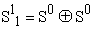,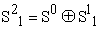and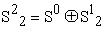in Figure 2). Assume that the theorem is valid whenever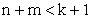. Let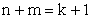. Let point x belong to G. Then the rim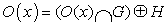of x in M is the join of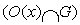and H, whereis the rim of x in G.is a digital (n-1)-surface by definition 3. Since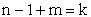thenis a digital (n+m)-surface by the induction hypothesis. Therefore, M is a digital (n+m)-surface by definition 3. Let point x belong to H. For the same reason as above,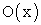is a digital (n+m)-surface. This completes the proof.

• Figure 1. Contractible graphs with the number of points n<5

Figure 2 illustrates the join of surfaces.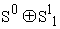and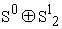are digital 2-dimensional surfaces, namely digital 2-dimensional spheres depicted in Figure 3.

• Figure 2. Digital 0-sphere S0. Digital 1-spheres S11, S12, S13. The join S0⊕S11 and S0⊕S12
• Figure 3. S21-S25 are digital 2-spheres. S3 is a digital 3-sphere

Definition 4

Digital n-surfaces are called homeomorphic if they are homotopy equivalent.

Homeomorphism means that a digital n-surface G can be converted to a homeomorphic digital n-surface H by contractible transformations. For example, digital 2-surfaces S21-S25 in Figure 3 are homeomorphic, i.e, every surface can be transformed to S21 by contractible transformations.

### 4. Digital n-dimensional Spheres and Manifolds

In this section, we consider graphs that are digital n-dimensional surfaces with locally-spherical topology. Paper 11 shows that a graph theoretical model of a continuous closed n-dimensional manifold is a digital n-dimensional surface with a locally-spherical topology. This means that in various applications and theories involving orientable and non-orientable continuous closed n-dimensional manifolds, these surfaces can be replaced by their graph theoretical models. This allows to apply computational or numerical methods instead of analytical methods.

Definition 5

• A digital n-dimensional surface G is called a digital n-sphere,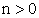, if for any point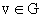, the rim O(v) is an (n-1)-sphere and the space G-v is a contractible graph (Figure 2- Figure 3).

• Let G be a digital n-sphere,, and v be a point belonging to G. The space D=G-v is called a digital n-disk with the boundary ∂D=O(v) and the interior IntD=D-∂D.

Graphs S11, S12 and S13 in Figure 2 are digital 1-spheres, graphs S21-S25 depicted in Figure 3 are digital 2-spheres, graph S3 if Figure 3 is a digital 3-sphere. The following results were obtained in 4, 6, 8.

Theorem 2

• Let G and H be digital n- and m-dimensional surfaces. Then the joinis a digital (n+m+1)-dimensional sphere if and only if G and H are digital n- and m-dimensional spheres

• The join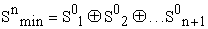of (n+1) copies of the zero-dimensional sphere S0 is a minimal n-sphere.

• Any n-sphere M can be converted to the minimal n-sphere Smin by contractible transformations.

It is easy to check directly thatin Figure 2,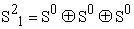and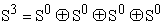in Figure 3; every 2-sphere depicted in Figure 3 can be converted into S21 by contractible transformations. In classical topology, a continuous n-dimensional sphere is a special case of a continuous n-dimensional manifold. Now, we outline a correspondence between continuous and digital n-dimensional manifolds.

Definition 6

A digital n-dimensional surface G is a digital n-manifold if for each point v of G, O(v) is a digital (n-1)-dimensional sphere (T and P in Figure 4).

• Figure 4. T is a digital torus. P is a digital projective plane. K is a digital Klein bottle. M is a digital Moebius band. Graphs T, P, K and M are obtained by identifying gray points with equal numbers

Evidently, a digital n-sphere is a digital n-manifold. The only difference between the digital n-sphere S and the digital n-manifold-non-sphere G is that for any point v, S-v is a contractible graph but G-v is not a contractible graph. In Figure 4, T is a digital torus. P is a digital projective plane. K is a digital Klein bottle. M is a digital Moebius band. All these graphs are obtained by identifying gray points with equal numbers. One can check directly that graphs T-v, P-v and K-v are not contractible. For example, P-v is homotopy equivalent to 1-sphere S11 depicted in Figure 2. The following statement is a consequence of definitions 3, 5 and 6.

Theorem 3

a) A digital 1-surface is a digital 1-sphere.

b) A digital 2-surface is a digital 2-manifold.

Proof.

a) To prove a), consider 1 digital 1-surface S13 in Figure 2. The rim O(x) of point x in S13 is a digital 0-sphere consisting of two non-adjacent points y and z. Therefore, x is adjacent to points y and z. S13 is connected and contains a finite number of points. By construction, S13-x is a contractible graph. Then S13 is a digital 1-sphere by definition 5.

b) To prove b) notice that if G is a digital 2-surface then the rim O(x) of a point x in G is a digital 1-surface by definition 3. Therefore, O(x) is a digital 1-sphere according theorem 4a). By definition 6, G is a digital 2-manifold. This completes the proof.

Thus for n=1,2, a digital n-surface is a digital n-manifold, i.e., the rim O(v) of every point v is a digital (n-1)-sphere. It is not true for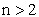as it is shown below.

### 5. Digital n-dimensional Surfaces with Local Non-spherical Topology

As it follows from previous section, if G is a digital n-manifold, then the rim O(v) of any point v is a digital (n-1)-sphere. However, there are digital n-dimensional surfaces, in which the rim of some or all points is different from a digital (n-1)-sphere. For example, the rim of some point can be a digital 2-dimensional torus, or a 2-dimensional projective plane, etc. To our knowledge, this kind of spaces has no analog in classical topology.

Definition 7

Let G be a digital n-dimensional surface. We say that:

• G is a digital n-dimensional surface with a locally spherical topology if for any point v in G, the rim O(v) of v is a digital (n-1)-dimensional sphere.

• G is a digital n-dimensional surface with a locally non-spherical topology if there is some point v in G such that the rim O(v) of v is a digital (n-1)-dimensional surface different from a digital (n-1)-sphere.

According to this definition and definition 6, a digital n-surface with a locally spherical topology is a digital n-manifold. As shown in 11, the graph theoretical model of a continuous closed n-dimensional manifold is necessarily a digital n-dimensional manifold. Several questions arise from this result, like what objects can be continuous counterparts of digital n-dimensional surfaces with a locally non-spherical topology.

Theorem 4

a) If G is a digital n-surface with locally non-spherical topology then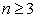.

b) Let G and H be digital n- and m-dimensional surfaces. Then the joinis a digital (n+m+1)-dimensional surface with locally non-spherical topology if H (or/and G) is not a digital m-sphere.

Proof

a) If G is a digital n-surface with locally non-spherical topology then there is a point x in G such that the rim O(x) is a digital (n-1)-surface, which is not a digital (n-1)-sphere. According to theorem 3, if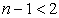then O(x) is a digital (n-1)-sphere. Therefore, if G is a digital n-surface with locally non-spherical topology then.

b) To prove b), use the induction on the dimension n. Suppose that n=0, i.e., G is a digital 0-sphere S(x,y). Then the rim of points x and y in M is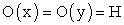, i.e., a digital m-manifold different from an m-sphere. Therefore, M is a (m+1)-dimensional surface with locally non-spherical topology by definition 7. Assume that the theorem is valid whenever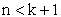. Let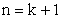. Let point x belong to G. Then the rim O(x) of x in M is, i.e., the join ofand H, whereis the rim of x in G, and a digital (n-1)-surface by definition 3. Since n-1+m=k then O(x) is a digital (n+m)-surface with locally non-spherical topology by the induction hypothesis. Therefore, M is a digital (n+m+1)-surface with locally non-spherical topology by definition 7. This completes the proof.

Consider examples of digital n-dimensional surfaces with a locally non-spherical topology.

Example 1 The join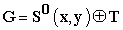of a digital 0-sphere S0 and a digital torus T

Let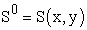be a digital 0-sphere, and let T be a digital torus containing sixteen points in Figure 5. Graph T is obtained by identifying points intersecting red and blue arrows respectively ( 6, 14). By theorem 1, graph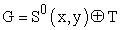is a digital 3-dimensional surface with eighteen points shown in Figure 5. In G, the rim of points x and y is torus T, O(x)=O(y)=T. If a point v belongs to T then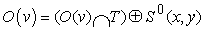. Since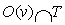is a digital 1-sphere S1 then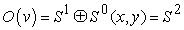is a digital 2-sphere by theorem 2. Thus, G is a digital 3-dimensional surface with a locally non-spherical topology of points x and y, because the rims O(x) and O(y) are both a digital 2-dimensional torus. One can say that points x and y have a toroidal local topology, all other points have a spherical topology.

• Figure 5. Graph T obtained by identifying points intersecting red and blue arrows respectively is a digital 2-torus. The join S0(x,y)⊕T is the digital 3-surface with a local non-spherical topology. The rims O(x) and O(y) of points x and y are both a digital 2-torus T. To make this operation visible, the join of a continuous torus T and S0(x,y) is shown

Example 2 The join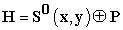of a digital 0-sphere S0 and a digital projective plane P

In Figure 6, graph P obtained by identifying red and blue points respectively is a digital 2-dimensional projective plane 6, 14. The join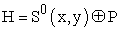is the digital 3-surface. The rims O(x) and O(y) of points x and y are both a digital 2-dimensional projective plane P. If a point v belongs to P then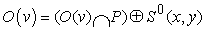. Since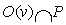is a digital 1-sphere S1 thenis a digital 2-sphere as it is in example 4.1. Thus, H is a digital 3-dimensional surface with a locally non-spherical topology, because the rims O(x) and O(y) are both a digital 2-dimensional projective plane P.

• Figure 6. Graph P obtained by identifying red and blue points respectively is a digital 2-dimensional projective plane. The join S0(x,y)⊕P is the digital 3-surface with a local non-spherical topology. The rims O(x) and O(y) of points x and y are both a digital 2-dimensional projective plane P. To make this operation visible, the join of a continuous projective plane P and S0(x,y) is shown

Apparently, H has no continuous counterparts in classical topology.

Example 3 The join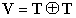of two digital tori T

The join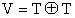is the digital homogeneous 5-dimensional surface depicted in Figure 7. For every point v belonging to V, the rim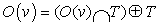. Sinceis a digital 1-sphere S1 then O(v) is a digital 4-dimensional surface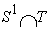. Evidently, O(v) is not a sphere. Therefore, the local topology of every point is non-spherical. It seems that this space, as spaces in previous examples, has no continuous counterparts in classical topology.

• Figure 7. Graph T obtained by identifying points intersecting red and blue arrows respectively is a digital 2-torus.T. The join T⊕T is the digital homogeneous 5-dimensional surface with a local non-spherical topology. To make this operation visible, the join of a continuous tori T is shown

### 6. Conclusion

This paper investigates the structure of graphs that are digital n-dimensional spaces with a locally non-spherical topology. It is proven that these spaces have the dimension. We show that the join of two digital tori is a digital homogeneous 5-dimensional surface with a local toroidal topology. Evidently, this type of spaces have no direct continuous counterparts among n-dimensional manifolds in classical topology.

We end with the problems for further study:

• What are continuous objects in classical topology that can be continuous analogs of digital n-dimensional surfaces with a locally non-spherical topology?

• What physical, chemical or biological structure can be described by digital n-dimensional surfaces with a locally non-spherical topology.

• It could be interesting to investigate general properties of digital n-dimensional surfaces with a locally non-spherical topology, such as the Euler characteristics, the homology groups, ets.

### References

  Cao, Z., Gu Cao, H.. Unified Field Theory and Topology of Nuclei, International Journal of Physics, 2(1), (2014). 15-22. In article View Article  Daragon, X., Couprie, M., Bertrand, G.. Discrete surfaces and frontier orders. Journal of Mathematical Imaging and Vision, 23 (3), (2005). 379-399. In article View Article  Eckhardt, U., Latecki, L.. Topologies for the digital spaces Z2 and Z3, Computer Vision and Image Understanding, 90, (2003). 295-312. In article View Article  Evako, A., Kopperman, R., Mukhin, Y.. Dimensional properties of graphs and digital spaces, Journal of Mathematical Imaging and Vision, 6, (1996). 109-119. In article View Article  Evako, A., Topological properties of closed digital spaces. One method of constructing digital models of closed continuous surfaces by using covers, Computer Vision and Image Understanding, 102, (2006). 134-144. In article View Article  Evako, A., Classification of digital n-manifolds, Discrete Applied Mathematics, 181, (2015). 289-296. In article View Article  Evako, A., Topology Preserving Discretization Schemes for Digital Image Segmentation and Digital Models of the Plane, Open Access Library Journal, 1: e566, (2014). In article View Article  Evako, A.. Topological properties of the intersection graph of covers of n-dimensional surfaces, Discrete Mathematics, 147, (1995). 107-120. In article View Article  Evako, A.. Characterizations of simple points, simple edges and simple cliques of digital spaces: One method of topology-preserving transformations of digital spaces by deleting simple points and edges, Graphical Models, 73 (2011) 1-9. In article View Article  Evako, A.. Dimension on discrete spaces, International Journal of Theoretical Physics, 33(7), (1994). 1553-1568. In article View Article  Evako, A.. Graph Theoretical Models of Closed n-Dimensional Manifolds: Digital Models of a Moebius Strip, a Projective Plane a Klein Bottle and n-Dimensional Spheres, International Journal of Discrete Mathematics, 2(3), (2017). 88-94. In article View Article  Gudder, S.. The Elementary Particle Cube, arXiv:1703.06023v1 [physics.gen-ph] Mar 2017. In article View Article  Ivashchenko, A.. Contractible transformations do not change the homology groups of graphs, Discrete Math., 126, (1994). 159-170. In article View Article  Ivashchenko, A.. Representation of smooth surfaces by graphs. Transformations of graphs which do not change the Euler characteristic of graphs, Discrete Math., 122, (1993). 219-233. In article View Article  Lu, W., and Wu, F.. Ising model on nonorientable surfaces: Exact solution for the Moebius strip and the Klein bottle, Phys. Rev., E 63, (2001). 026107. In article View Article  PubMed  Segonne, F., Fischl, B.. Integration of Topological Constraints in Medical Image Segmentation. Handbook of Biomedical Imaging: Methodologies and Clinical Research, 245-262, (2014). In article PubMed  PubMedThis work is licensed under a Creative Commons Attribution 4.0 International License. To view a copy of this license, visit http://creativecommons.org/licenses/by/4.0/